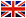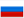X-ray structural analysis

S.V. Simonov

Annotation

The course is aimed to acquaint the students with the basic principles of the X-Ray structural analysis. The most important concepts of the modern crystallography, crystal symmetry and methods of crystal structure determination are considered. Physical base of interaction of X-rays, electrons and slow neutrons with material is formulated. The main experimental aims of X-ray diffraction study of classic crystal structure and real structure are analyzed. The special attention is given to recently obtained objects – quasicrystals, modulated structures, fullerenes.

Program
I. Elements of crystallography. Theory of crystal lattice.
1. Translation. Crystallographic plane. Unit cell. Lattice parameters. Symmetry operations. Symmetry elements.
2. Combinations of symmetry elements. Generation of symmetry elements. Crystallographic point groups. Crystal systems. Matrix representation of symmetry operations and point groups. Curie groups. Point groups of ikosahedral symmetry. Quasicrystals.
3. Space groups. Choice of unit cell. The 14 Bravais lattices. Comparison of concepts — a crystal lattice and atomic crystal structure.
4. Translation as symmetry element. Screw axes and glide planes. Matrix representation of translational symmetry elements. Space groups symbols according to the international system.
5. Special and general positions in crystal lattice. Relation between multiplicity and type of position. Full description of known crystal structure. Close-packed structures. Defects of packing. Tetrahedral and octahedral voids in the structure.
6. Crystal polyhedrons. Simple crystal forms. The Platon's figures as simple forms of cubic and icosahedral symmetry. Crystal lattice. Types of crystallographic projections — linear, gnomonic, stereographic, gnomostereographic. The Wulf net.
7. Metrics of reciprocal space. Relations between reciprocal lattice and direct crystal lattice.

II. Generation of X-rays and their interaction with material.
8. X-ray equipment. Types of X-ray tubes.
9. X-ray spectrum.
10. Synchrotron radiation: physical principles and applications.
11. X-ray scattering and absorption in material. Linear absorption factors: its dependence on wave length and atomic number. Selective absorption and filters.
12. Scattering by a free electron. Thomson formula. Atomic scattering factors. Calculation of atomic scattering amplitudes.

III. X-ray diffraction in a crystal.
13. The "geometrical" theory of diffraction on a three-dimensional crystal lattice. Wulf-Bragg equation in vector and scalar expression. Ewald construction. Brillouin zone borders.
14. The main diffraction schemes in representation of a reciprocal lattice: Laue method, powder diffraction method, oscillation method, X-ray diffraction on powder sample and single crystal.
15. Intensity of X-ray diffraction maxima. Scattering by one unit cell. Structural amplitude: general expression.
16. Laue's interference function. Scattering by a small crystal. Conditions for diffraction maxima appearance. Shape and size of knots of reciprocal lattice.
17. Formula for calculation of reflection intensity. Integral factor. Diffraction from system of the planes. Thermal factor. Repetition factor. Angular factor.
18. Analysis of structural factors. The systematic absences for Bravais lattices, screw axes and glide planes.
19. Fourier synthesis as method of the crystal structure analysis. Structural amplitudes as factors of a Fourier row. Reciprocal lattice, weight of knots, geometrical image of Fourier transformation.

IV. The main goals of X-ray structural analysis.
20. Determination of new structure. Application of various experimental schemes for determination of crystal system, Bravais lattice type, point and space group symmetry, number of atoms in unit cell. Experimental and calculation methods of atomic position determination. Trial and error method. Patterson synthesis. Fourier synthesis.
21. Structural analysis of metallic systems. Phase identification on phase diagram. Ordering of solid solutions. Dispersive solidification in alloys by X-ray mapping.
22. Structural transformations and aperiodic crystals. Diffusionless Martensite transformations, polytypic crystals, incommensurate modulated structures, quasicrystals, fullerenes.

V. Electron and neutron diffraction.
23. Electron diffraction. Principle and scopes of fast electron diffraction method. The main goals of slow electron diffraction.
24. Neutron diffraction. Nuclear scattering: application and principles. Magnetic scattering, ordering of magnetic moments in structure of ferro- and antiferromagnets.

VI. X-ray diffraction microscopy.
25. Kinematic and dynamic theory of diffraction. Primary and secondary extinction. Ideal crystal. Mosaic crystal.
26. Analysis of real crystal structure. Berg-Barrett topography. Fudzivar, Schultz, angular scanning methods.
27. Methods of ideal crystal studies, using the phenomena within the dynamic theory. Lang scheme. Anomalous transmission effect, Borman method.

References
1. Modern Crystallography 1: Fundamentals of Crystals. Symmetry, and Methods of Structural Crystallography, Boris K. Vainshtein, Springer; 2nd edition, 2003.
2. X-ray crystallographic technology. Andr? Guinier, Hilger and Watts (Hilger Division), 1952Agarkov D.A. • Tel: +7(916)7584930 • email: agarkov@issp.ac.ru